Powered by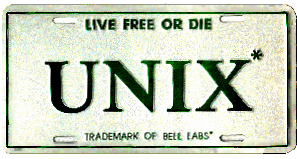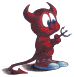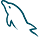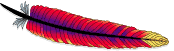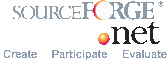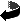Up
 Two forces govern the movement. Coulomb force (qV/d) Newton force (mg)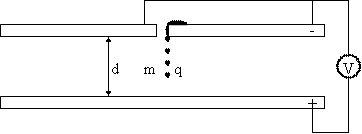q = droplet charge; V = voltage between plates; d = distance between plates; m = droplet mass; g = let's take as standard gravity